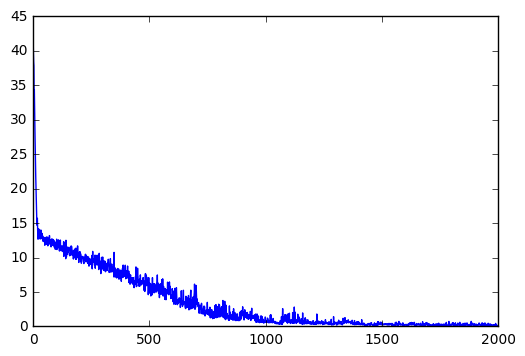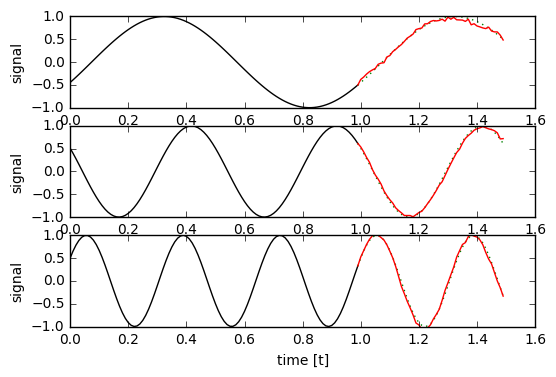# Caffe LSTM Example on Sin(t) Waveform Prediction¶

### with Mini-Batch Training¶

I used to create LSTM networks using plain Python/Numpy or using Tensorflow. Recently I started to use Caffe, which is a wonderful framework, but has terrible documents for beginners to pick it up. I tried to create LSTM networks in Caffe and got lots of issues. There are many LSTM examples in Tensorflow, but it is nearly a desert for Caffe LSTM examples. I searched on google, found several Caffe LSTM examples online, but I could not get any of those working. So I spent sometime studying Caffe code and then adapted Tensorflow Sin(t) example to Caffe.

In :
import numpy as np
import math
import os
import caffe
import matplotlib
import matplotlib.pyplot as plt
%matplotlib inline

GPU_ID = 0
caffe.set_mode_gpu()
caffe.set_device(GPU_ID)


In :
# Use the sample generator from Tensorflow Sin(t) online
def generate_sample(f = 1.0, t0 = None, batch_size = 1, predict = 50, samples = 100):
"""
Generates data samples.

:param f: The frequency to use for all time series or None to randomize.
:param t0: The time offset to use for all time series or None to randomize.
:param batch_size: The number of time series to generate.
:param predict: The number of future samples to generate.
:param samples: The number of past (and current) samples to generate.
:return: Tuple that contains the past times and values as well as the future times and values. In all outputs,
each row represents one time series of the batch.
"""
Fs = 100.0

T = np.empty((batch_size, samples))
Y = np.empty((batch_size, samples))
FT = np.empty((batch_size, predict))
FY = np.empty((batch_size, predict))

_t0 = t0
for i in range(batch_size):
t = np.arange(0, samples + predict) / Fs
if _t0 is None:
t0 = np.random.rand() * 2 * np.pi
else:
t0 = _t0 + i/float(batch_size)

freq = f
if freq is None:
freq = np.random.rand() * 3.5 + 0.5

y = np.sin(2 * np.pi * freq * (t + t0))

T[i, :] = t[0:samples]
Y[i, :] = y[0:samples]

FT[i, :] = t[samples:samples + predict]
FY[i, :] = y[samples:samples + predict]

return T, Y, FT, FY

In :
solver = caffe.AdamSolver('Caffe_TOY_LSTM_batch_solver.prototxt')

In :
# Network Parameters
n_input = 1 # single input stream
n_steps = 100 # timesteps
n_hidden = 150 # hidden units in LSTM
n_outputs = 50 # predictions in future time
batch_size = 20 # batch of data

In :
# Train network
niter = 2000
disp_step = 200
train_loss = np.zeros(niter)
solver.net.params['lstm1'].data[n_hidden : 2 * n_hidden] = 1
solver.net.blobs['clip'].data[...] = 1
#solver.net.blobs['clip'].data = 0
for i in range(niter):
_, batch_x, _, batch_y = generate_sample(f=None,
t0=None,
batch_size=batch_size,
samples=n_steps,
predict=n_outputs)
batch_x = batch_x.transpose()
#batch_y = batch_y.transpose()
solver.net.blobs['label'].data[:,:] = batch_y
solver.net.blobs['data'].data[:,:,0]  = batch_x
solver.step(1)
train_loss[i] = solver.net.blobs['loss'].data
if i % disp_step == 0:
print "step ", i, ", loss = ", train_loss[i]
print "Finished training, iteration reached", niter

step  0 , loss =  41.7146110535
step  200 , loss =  9.77667808533
step  400 , loss =  7.38890314102
step  600 , loss =  4.39145421982
step  800 , loss =  1.36509764194
step  1000 , loss =  0.663882374763
step  1200 , loss =  0.351818472147
step  1400 , loss =  0.291823685169
step  1600 , loss =  0.251198112965
step  1800 , loss =  0.189343497157
Finished training, iteration reached 2000

In :
# plot loss value during training
plt.plot(np.arange(niter), train_loss)

Out:
[<matplotlib.lines.Line2D at 0x7f85acce8590>]In :
# Test the prediction with trained net
n_tests = 3
solver.test_nets.blobs['data'].reshape(n_steps, 1, 1)
solver.test_nets.blobs['clip'].reshape(n_steps, 1)
solver.test_nets.reshape()

for i in range(1, n_tests + 1):
plt.subplot(n_tests, 1, i)
t, y, next_t, expected_y = generate_sample(f=i, t0=None, samples=n_steps, predict=n_outputs)
test_input = y.transpose()
expected_y = expected_y.reshape(n_outputs)
solver.test_nets.blobs['clip'].data[...] = 1
solver.test_nets.blobs['data'].data[:,:,0]  = test_input
solver.test_nets.forward()
prediction = solver.test_nets.blobs['ip1'].data

# remove the batch size dimensions
t = t.squeeze()
y = y.squeeze()
next_t = next_t.squeeze()
prediction = prediction.squeeze()

plt.plot(t, y, color='black')
plt.plot(np.append(t[-1], next_t), np.append(y[-1], expected_y), color='green', linestyle=":")
plt.plot(np.append(t[-1], next_t), np.append(y[-1], prediction), color='red')
plt.ylim([-1, 1])
plt.xlabel('time [t]')
plt.ylabel('signal')
plt.show()Published: January 30 2018

• category: